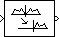# Analytic Signal

Compute analytic signals of discrete-time inputs

## Library

Transforms

`dspxfrm3`

•## Description

The Analytic Signal block computes the complex analytic signal corresponding to each channel of the real M-by-N input, u

`$y=u+jH\left\{u\right\}$`

where $j=\sqrt{-1}$ and H{ } denotes the Hilbert transform. The real part of the output in each channel is a replica of the real input in that channel; the imaginary part is the Hilbert transform of the input. In the frequency domain, the Fourier transform of the analytic signal doubles the positive frequency content of the original signal while zeroing-out negative frequencies and retaining the DC component.

The block computes the Hilbert transform using an equiripple FIR filter with the order specified by the Filter order parameter, n. The linear phase filter is designed using the Remez exchange algorithm, and imposes a delay of n/2 on the input samples.

The output has the same dimensions as the input.

This block supports SIMD code generation. For details, see Code Generation.

### Frame-Based Processing

When you set the Input processing parameter to `Columns as channels (frame based)`, the block performs frame-based processing. In this mode, the block treats an M-by-N matrix input as N independent channels containing M sequential time samples. The block computes the analytic signal for each channel over time.

### Sample-Based Processing

When you set the Input processing parameter to `Elements as channels (sample based)`, the block performs sample-based processing. In this mode, the block treats an M-by-N matrix input as M*N independent channels and computes the analytic signal for each channel (matrix element) over time.

## Parameters

Filter order

The length of the FIR filter used to compute the Hilbert transform.

Input processing

Specify how the block should process the input. You can set this parameter to one of the following options:

• `Columns as channels (frame based)` (default) — When you select this option, the block treats each column of the input as a separate channel.

• `Elements as channels (sample based)` — When you select this option, the block treats each element of the input as a separate channel.

## Supported Data Types

• Double-precision floating point

• Single-precision floating point

## Version History

Introduced before R2006a